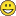# QlikView App Dev

Discussion Board for collaboration related to QlikView App Development.

Announcements
Skip the ticket, Chat with Qlik Support instead for instant assistance.
cancel
Showing results for
Did you mean:Anonymous
Not applicable

## Sum over a calculation + storing in variable

Let's hope this won't get too complicatedSo imagine that you have a dimension which is just a customer number. Each customer has orders with an order date. You need the first and the last order date, the others are not important. Using min(field name for date) and max(field name for date) does the trick for me.

Based on these min and max values, each customer will be assigned two binary values. The first one is the denominator (I just call it that, you will see why soon). If the first order falls in a given time period, this value becomes 1, otherwise it is 0. So you get a column of 1's and 0'. Note that this calculation is made using variables that store time elements to construct a time interval. And if the first order is in that period, the customer gets a 1.

For the numerator the process is the same. If the denominator is 1, the numerator can become 1 if and only if the last order falls in a certain time period.

Now add an extra column that gives the country a person lives in so that you have a customer id, the nominator and denominator and the country a person lives in.

I need a total of 4 variables I think for the calculations so you can change the values off those and it will give different 1's and 0'.

Example:

Print1.png

So up to this point everything goes well. Now, I want to be able to sum the numerator, the denominator (Total sum option is grey and partial sums give me 0 so am I doing something wrong or should I force the 1 and 0 to be num? I just use if(expression,1,0)), then divide them (so N/D) and then do this for every country so I get a % per country.

1. How do I do this? Because it is summing over the expression and THEN dividing the sum of both OVER the country (I assume it has to do with aggr function, pivot table with double dimension: customer and country, something like that?

2. Could it be possible to use a variable to store the total result in? If not then that is fine but I do have another question related to this.

I know it is possible to use a variable in a set analysis. However, is it possible to use a set expression in a variable?

For example, if I am able to let's say store the TOTAL % over all countries (would be kinda hard to store each countries % in a different variable), could it be doable using a set analysis expression where you can use another variable to change the set expression's search range? Example:

I want to calculate that % so the sum of all 1's in numerator divided by sum of all 1's in the denominator gives me a % for all customers but I want to change my variables so the I get another time frame to calculate the 1's and 0's and then the values should change accordingly.

I hope this explanation is clear enough and that you don't need the qvw file to simulate this. If there are any questions just let me know and I'll try to explain them or answer them.

Big thanks in advance1 Solution

Accepted SolutionsMVP

I think what you need is this

Sum of rows in pivot tables

Sum(Aggr(If(expression,1,0), Customer, Country))

or this

Sum(TOTAL <Customer> Aggr(If(expression,1,0), Customer, Country))

2 RepliesMVP

I think what you need is this

Sum of rows in pivot tables

Sum(Aggr(If(expression,1,0), Customer, Country))

or this

Sum(TOTAL <Customer> Aggr(If(expression,1,0), Customer, Country))Anonymous
Not applicable
Author

YesThat is indeed what I was looking for. I knew it was the aggr function but I just can't work with it that well hahaThank you (once again, you've helped me quite a bit!)!!!

Kind regardsCommunity Browser